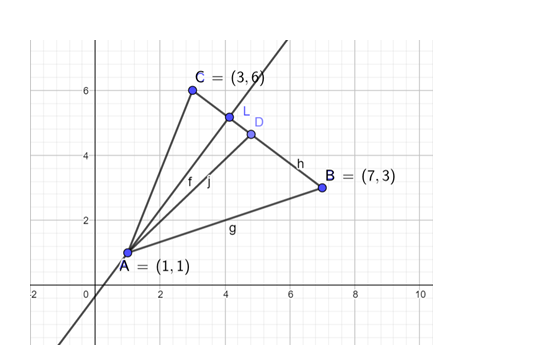Deepak Scored 45->99%ile with Bounce Back Crack Course. You can do it too!

# A(1, 1), B(7, 3) and C(3, 6) are the vertices of a ΔABC

Question:

A(1, 1), B(7, 3) and C(3, 6) are the vertices of a ΔABC. If D is the midpoint of BC and AL ⊥ BC, find the slopes of

(ii) AL.

Solution:Given points are

A(1, 1), B(7, 3) and C(3, 6)

slope $=\left(\frac{y_{2}-y_{1}}{x_{2}-x_{1}}\right)$

Slope of line $B C=\left(\frac{3-6}{7-3}\right)=\frac{-3}{4}$

(i) As $D$ is the midpoint of $B C$, coordinate of $D$ are $D\left(\frac{x_{1}+x_{2}}{2}, \frac{y_{1}+y_{2}}{2}\right)$

$=\left(\frac{7+3}{2}, \frac{3+6}{2}\right)=\left(5, \frac{9}{2}\right)$

Now the slope of $A D=\left(\frac{9}{2-1}\right)=\left(\frac{7}{2}\right)=\frac{3.5}{4}$

(ii) As $\mathrm{AL}$ is perpendicular to $\mathrm{BC}$

The slope of $A L \times$ slope of $B C=-1$

Let slope of $\mathrm{AL}$ be $\mathrm{m}_{1}$

$\frac{-3}{4} \times m_{1}=-1$

$\Rightarrow \mathrm{m}_{1}=\frac{4}{3}$

So Slope of line $A L$ is $\frac{4}{3}$.